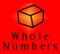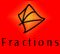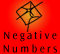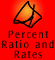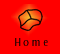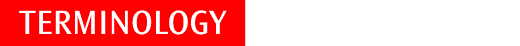| Base ten | Common fraction |
| Commutative Law of Multiplication | Decimal numbers |
| Partition | Quotition |

Base Ten/Decimal Place Value System

The word decimal comes from the Latin word decimus meaning tenth. Deca means 10 and comes from the Latin word decem (ten) and the Greek word deka.

The decimal place value system then, is a way of counting and recording numbers using ten as a base for grouping.

All numbers in the decimal system are made up from ten digits:

0 1 2 3 4 5 6 7 8 9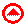Common Fraction

A common (or vulgar) fraction is way of writing numbers as a fraction whose numerator and denominator are both whole numbers.

A fraction represents the ratio of two numbers.

A fraction is the result obtained by dividing one number by another.Commutative Property of Multiplication

The order in which two or more numbers are multiplied does not affect the answer (product).

This property can be expressed as:

a x b = b x a

An array model demonstrates this property of multiplication:

 * * * * * *

is the same as

 * * * * * *

The array model demonstrates the property for integers, but it holds for fractions and decimals too.Decimal numbers

A decimal number is a number which is written using the base ten place value system and a decimal point. Decimal numbers are written without denominators, using the decimal point to indicate the position of the ones column:

2/10 = 0.2, 2/100 = 0.02, 2/1000 = 0.002, 2/10 000 = 0.0002

Decimal fractions

Another name for decimal numbers.Partition division

Partition division situations involve sharing a quantity into a given number of parts.

For example, we are sharing 24 cakes between 3 people. How many cakes will each person receive?Quotition division

Quotition division involves dividing a number into given quotas.

For example: Twenty four cakes were shared equally amongst the children and everyone got 3. How many children were there?